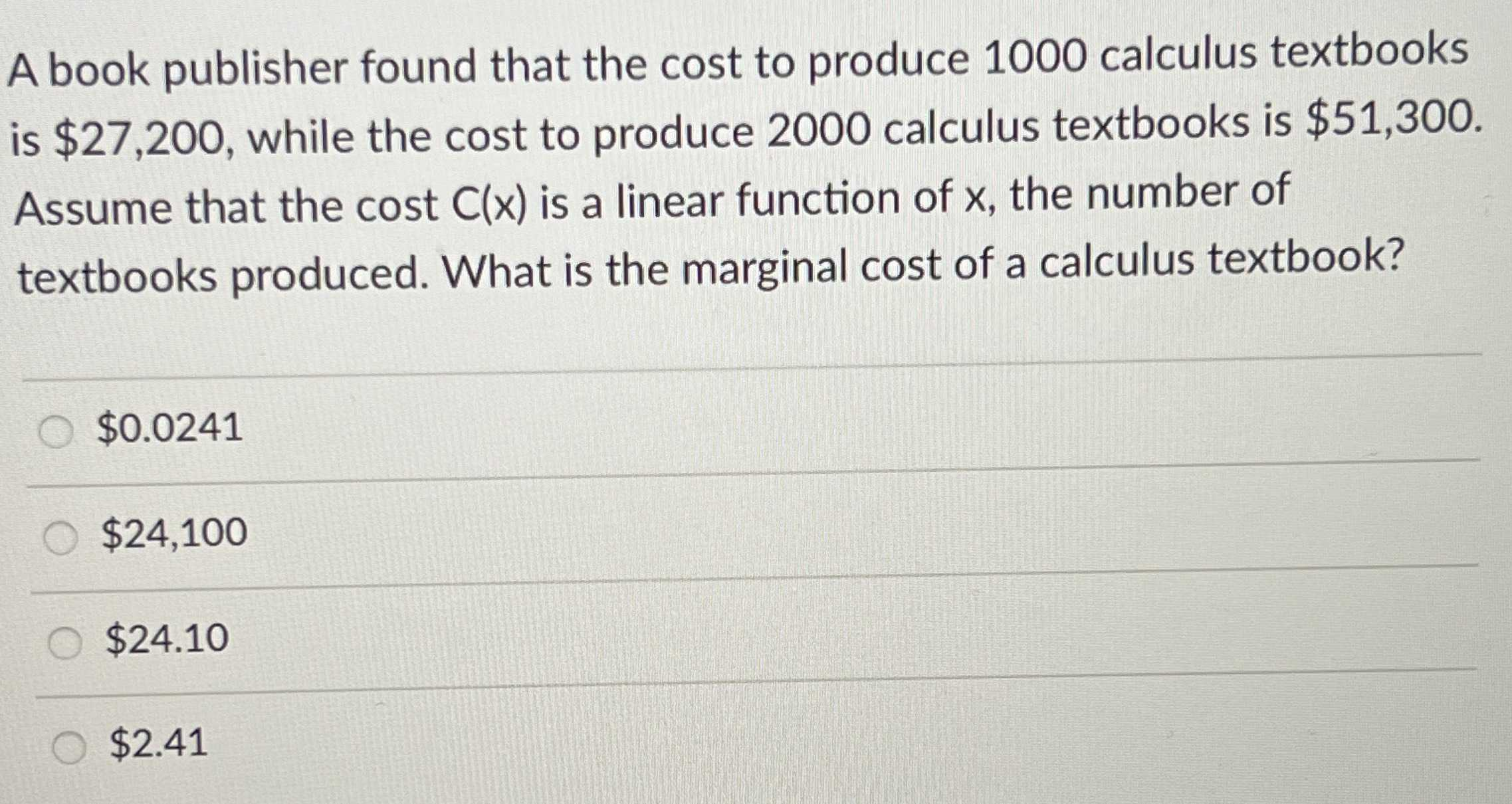### Still have math questions?

Algebra
QuestionA book publisher found that the cost to produce $$1000$$ calculus textbooks is $$\ 27,200$$ , while the cost to produce $$2000$$ calculus textbooks is $$\ 51,300$$ . Assume that the cost $$C ( x )$$ is a linear function of $$x$$ , the number of textbooks produced. What is the marginal cost of a calculus textbook?

$$\ 0.0241$$

$$\ 24,100$$

$$\ 24.10$$

$$\ 2.41$$